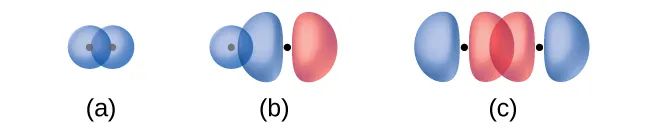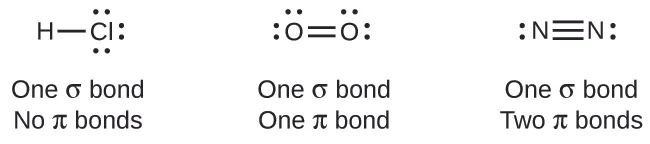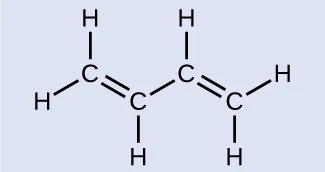# 11

Derived from Valence Bond Theory by OpenStax

### Atomic Orbital Overlap

There are successful theories that describe the electronic structure of atoms. We can use quantum mechanics to predict the specific regions around an atom where electrons are likely to be located: A spherical shape for an s orbital, a dumbbell shape for a p orbital, and so forth. However, these predictions only describe the orbitals around free atoms. When atoms bond to form molecules, atomic orbitals are not sufficient to describe the regions where electrons will be located in the molecule. A more complete understanding of electron distributions requires a model that can account for the electronic structure of molecules. One popular theory holds that a covalent bond forms when a pair of electrons is shared by two atoms and is simultaneously attracted by the nuclei of both atoms. In the following sections, we will discuss how such bonds are described by valence bond theory and hybridization.

Valence bond theory describes a covalent bond as the overlap of half-filled atomic orbitals (each containing a single electron) that yield a pair of electrons shared between the two bonded atoms. We say that orbitals on two different atoms overlap when a portion of one orbital and a portion of a second orbital occupy the same region of space. According to valence bond theory, a covalent bond results when two conditions are met: (1) an orbital on one atom overlaps an orbital on a second atom and (2) the single electrons in each orbital combine to form an electron pair. The mutual attraction between this negatively charged electron pair and the two atoms’ positively charged nuclei serves to physically link the two atoms through a force we define as a covalent bond. The strength of a covalent bond depends on the extent of overlap of the orbitals involved. Orbitals that overlap extensively form bonds that are stronger than those that have less overlap.

In addition to the distance between two orbitals, the orientation of orbitals also affects their overlap (other than for two s orbitals, which are spherically symmetric). Greater overlap is possible when orbitals are oriented such that they overlap on a direct line between the two nuclei. Figure 1 illustrates this for two p orbitals from different atoms; the overlap is greater when the orbitals overlap end to end rather than at an angle.(a) The overlap of two p orbitals is greatest when the orbitals are directed end to end. (b) Any other arrangement results in less overlap. The dots indicate the locations of the nuclei.

The overlap of two s orbitals (as in H2), the overlap of an s orbital and a p orbital (as in HCl), and the end-to-end overlap of two porbitals (as in Cl2) all produce sigma bonds (σ bonds), as illustrated in Figure. A σ bond is a covalent bond in which the electron density is concentrated in the region along the internuclear axis; that is, a line between the nuclei would pass through the center of the overlap region. Single bonds in Lewis structures are described as σ bonds in valence bond theory.Sigma (σ) bonds form from the overlap of the following: (a) two s orbitals, (b) an s orbital and a p orbital, and (c) two p orbitals. The dots indicate the locations of the nuclei.

pi bond (π bond) is a type of covalent bond that results from the side-by-side overlap of two p orbitals, as illustrated in Figure. In a π bond, the regions of orbital overlap lie on opposite sides of the internuclear axis. Along the axis itself, there is a node, that is, a plane with no probability of finding an electron.Pi (π) bonds form from the side-by-side overlap of two p orbitals. The dots indicate the location of the nuclei.

While all single bonds are σ bonds, multiple bonds consist of both σ and π bonds. As the Lewis structures below suggest, O2 contains a double bond, and N2 contains a triple bond. The double bond consists of one σ bond and one π bond, and the triple bond consists of one σ bond and two π bonds. Between any two atoms, the first bond formed will always be a σ bond, but there can only be one σ bond in any one location. In any multiple bond, there will be one σ bond and the remaining one or two bonds will be π bonds. These bonds are described in more detail later in this chapter.Counting σ and π BondsButadiene, C4H6, is used to make synthetic rubber. Identify the number of σ and π bonds contained in this molecule.

SolutionThere are six σ C–H bonds and one σ C–C bond, for a total of seven from the single bonds. There are two double bonds that each have a π bond in addition to the σ bond. This gives a total nine σ and two π bonds overall.

Identify each illustration as depicting a σ or π bond:

(a) side-by-side overlap of two p orbitals                                      (b) end-to-end overlap of two p orbitals(a) is a π bond with a node along the axis connecting the nuclei while (b) is σ bonds that overlap along the axis.

### Key Concepts and Summary

Valence bond theory describes bonding as a consequence of the overlap of two separate atomic orbitals on different atoms that creates a region with one pair of electrons shared between the two atoms. When the orbitals overlap along an axis containing the nuclei, they form a σ bond. When they overlap in a fashion that creates a node along this axis, they form a π bond. Dipole moments can be used to determine partial separations of charges between atoms.

### Chemistry End of Chapter Exercises

1.  Explain how σ and π bonds are similar and how they are different.
2.   Use valence bond theory to explain the bonding in F2, HF, and ClBr. Sketch the overlap of the atomic orbitals involved in the bonds.

3.   Use valence bond theory to explain the bonding in O2. Sketch the overlap of the atomic orbitals involved in the bonds in O2.

4.   How many σ and π bonds are present in the molecule HCN?

5.   A friend tells you N2 has three π bonds due to overlap of the three p-orbitals on each N atom. Do you agree?

6.   Draw the Lewis structures for CO2 and CO, and predict the number of σ and π bonds for each molecule.

(a) CO2

(b) CO

### Glossary

overlap
coexistence of orbitals from two different atoms sharing the same region of space, leading to the formation of a covalent bond
node
plane separating different lobes of orbitals, where the probability of finding an electron is zero
pi bond (π bond)
covalent bond formed by side-by-side overlap of atomic orbitals; the electron density is found on opposite sides of the internuclear axis
sigma bond (σ bond)
covalent bond formed by overlap of atomic orbitals along the internuclear axis
valence bond theory
description of bonding that involves atomic orbitals overlapping to form σ or π bonds, within which pairs of electrons are shared